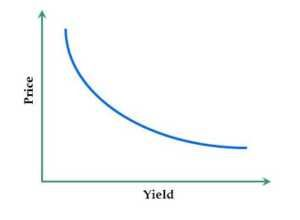# Yield Gap

The difference between the yields of government-issued securities and shares

## What is Yield Gap?

The Yield Gap is the difference between the yields of government-issued securities and the average dividend yield on stock shares. In other words, yield gap, or the yield gap ratio, is the ratio of the dividend yield on equity compared to the yield on long-term government bonds.

Yield gap is calculated to determine whether equity is underpriced or overpriced compared to government bonds. The smaller the yield gap, the lower is the equity yield compared to government bonds, indicating that equity is overpriced. Conversely, the higher the yield gap, the greater is the yield on equity compared to government bonds, indicating that equity is relatively underpriced.

### Reverse Yield Gap

The reverse yield gap is that amount by which the yield on bonds exceeds the yield on equity or, in other words, the amount by which the interest on loans and bonds exceeds the cost of equity. The reverse yield gap only applies during those time periods when the average yield from investing in bonds is higher than the average dividend yield on stocks.

### Interpretation of Yield Gap

A positive yield gap indicates more opportunities to buy in the equity markets, especially during periods of high inflation. However, when consumer prices are stable, a positive gap is not as strong an indicator because during periods of very low inflation, investors are more inclined to accept lower yields.

A widening gap between bond and equity yields indicates a new growth cycle.

### Yield on Equity

The Yield on Equity is the earnings or return on an investment’s equity portion. It is the amount of earnings compared to the amount invested in equity.

##### #1 Earnings Yield

Earnings yield is the Earning per Share (EPS) divided by the Market Price Per Share (MPS). Earnings yield shows the percentage of each unit of currency invested in the stock that was earned by a company.

##### #2 Dividend Yield

The dividend yield is a financial ratio that indicates the amount a company pays out in the form of dividends each year relative to the value of its shares or Market Price Per Share. In other words, the dividend yield is Dividend per Share (DPS) divided by Market Price per Share (MPS).

The dividend yield on stocks is the yield referred to in calculating the yield gap – not the total per share return on equity for a stock investment. The average dividend yield is usually estimated as the average dividend yield of a major stock index, such as the S&P 500 Index.

### Yield on Bond

Yield on Bond is the amount of return an investor realizes on a bond.  In other words, the yield on a bond is the amount an investor earns in the form of interest paid to them by the borrower or issuer of the bond.

Nominal Yield is the interest paid by the issuer of the bond divided by the face value of the bond. Current yield is the annual earnings of the bond divided by the current market price of the bond. Required yield is the minimum yield amount expected by investors as compensation for the risk of investing.As the price of a bond increases, the yield on bond falls; and as the price of a bond falls, the yield on bond increases.

### Other resources

• Investing: A Beginner’s Guide
• Bond Pricing
• Equity vs Fixed Income